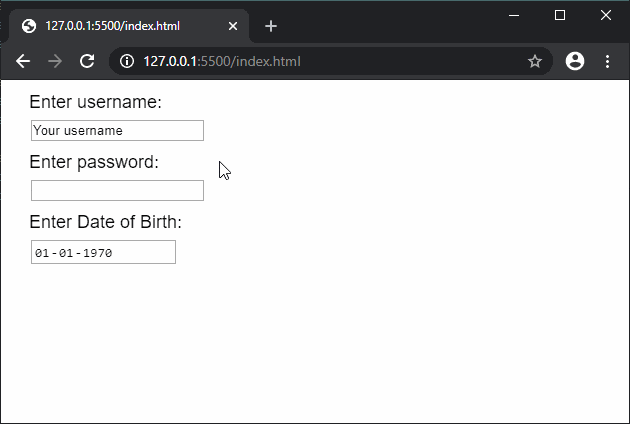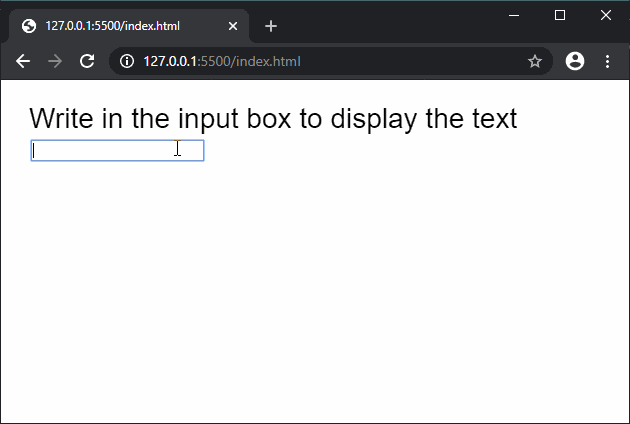# p5.js | createInput() Function

• Last Updated : 26 Feb, 2020

The createInput() function is used to create an input element in the DOM for accepting text input. The optional parameters can be used to set the type of input that can be entered. You can use the set() function to define the length of the box.

Syntax:

Hey geek! The constant emerging technologies in the world of web development always keeps the excitement for this subject through the roof. But before you tackle the big projects, we suggest you start by learning the basics. Kickstart your web development journey by learning JS concepts with our JavaScript Course. Now at it's lowest price ever!

`createInput(value, type)`

Parameters: This functione accepts two parameters as mentioned above and described below:

• value: This string parameter used to set the default value of the input.
• type: This is a string parameter which sets the type of input. It can have values such as ‘text’, ‘password’, etc for accepting text with that specific format.

Return Value: It returns a pointer to the p5.Element with the created node.

Below examples illustrate the createInput() function in p5.js:

Example 1:

 `function` `setup() {``  ``createCanvas(300, 200);``  ``textSize(18);`` ` `  ``text(``"Enter username:"``, 20, 20);``  ``usernameInput = createInput(``'Your username'``, ``'text'``);``  ``usernameInput.position(30, 40);`` ` `  ``text(``"Enter password:"``, 20, 80);``  ``passInput = createInput(``''``, ``'password'``);``  ``passInput.position(30, 100);`` ` `  ``text(``"Enter Date of Birth:"``, 20, 140); ``  ``dobInput = createInput(``'1970-01-01'``, ``'date'``);``  ``dobInput.position(30, 160);``}`

Output:Example 2:

 `function` `setup() {``  ``createCanvas(600, 300);``  ``textSize(28);``  ``text(``"Write in the input box to display the text"``, 20, 40);`` ` `  ``// Create input element``  ``let inputElem = createInput(``''``);``  ``inputElem.input(onInput);``  ``inputElem.position(30, 60)``}`` ` `function` `onInput() {``  ``clear();``  ``text(``"Write in the input box to display the text"``, 20, 40);`` ` `  ``fill(``"green"``)``  ``strokeWeight(10)``  ``rect(0, 100, 600, 100)`` ` `  ``// Display the text entered``  ``fill(``"black"``)``  ``text(``this``.value(), 20, 140)``}`

Output:Online editor: https://editor.p5js.org/

My Personal Notes arrow_drop_up